NEET  >  Test: Physics - 8

# Test: Physics - 8

Test Description

## 45 Questions MCQ Test NEET Mock Test Series | Test: Physics - 8

Test: Physics - 8 for NEET 2023 is part of NEET Mock Test Series preparation. The Test: Physics - 8 questions and answers have been prepared according to the NEET exam syllabus.The Test: Physics - 8 MCQs are made for NEET 2023 Exam. Find important definitions, questions, notes, meanings, examples, exercises, MCQs and online tests for Test: Physics - 8 below.
Solutions of Test: Physics - 8 questions in English are available as part of our NEET Mock Test Series for NEET & Test: Physics - 8 solutions in Hindi for NEET Mock Test Series course. Download more important topics, notes, lectures and mock test series for NEET Exam by signing up for free. Attempt Test: Physics - 8 | 45 questions in 45 minutes | Mock test for NEET preparation | Free important questions MCQ to study NEET Mock Test Series for NEET Exam | Download free PDF with solutions
 1 Crore+ students have signed up on EduRev. Have you?
Test: Physics - 8 - Question 1

### Two charges of magnitude Q and two charges of magnitude –q are placed at the opposite corners of a square of side length ‘a’. If the resultant force on a charge Q is zero than

Test: Physics - 8 - Question 2

### Workdone in carrying a charge ‘Q’ from ‘A’ to ‘B’ (as shwon in figure) on a circle of radius ‘r’ with a charge ‘Q’ at the centre is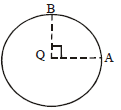Test: Physics - 8 - Question 3

### Two spheres having radii of 5 cm and 10 cm bear identical charge of 6.6 nC. Find the potential of spheres after they have been connected by a conductor, assume that the spheres are at large distance from each other

Test: Physics - 8 - Question 4
Two point charge 36 and –16 microcoulomb are placed 10 cm aptart. At a point X, there is no resultant force on unit positive charge. ‘X’ will lie somewhere
between (see the diagram)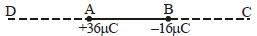Test: Physics - 8 - Question 5

If the surface density of charge be  σ electric field near the surface would be

Test: Physics - 8 - Question 6

A charged particle of mass 0.1 g is in equilibrium in an electric field E = 98 N/C. The charge on the particle is

Test: Physics - 8 - Question 7

An electric charge ‘q’ is lying at the open end of a cylindrical vessel. The flux coming out of the cylinder will be

Test: Physics - 8 - Question 8

The inward and outward electric flux for a closed surface in units of
N - m2/coulomb are respectively 8x103 and 4x103 Then the total charge inside the surface is

Test: Physics - 8 - Question 9

A bullet of mass 2g is having a charge of 2 μC.Through what potential difference must be accelerated, starting from rest, to acquire speed of 10 m/s?

Detailed Solution for Test: Physics - 8 - Question 9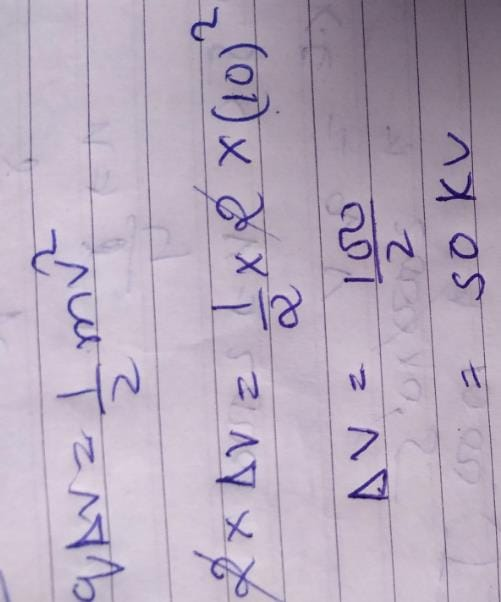Test: Physics - 8 - Question 10

On moving a charge of 20 coloumbs by 2cm, 2J of work is done, then the potential difference between the points is

Test: Physics - 8 - Question 11

The capacitance of a parallel plate condenser does not dpened upon

Test: Physics - 8 - Question 12

A semi-circular are of radius ‘a’ is charged uniformly and the charge per unit length is  λ The electric field at its centre is

Test: Physics - 8 - Question 13
A parallel plate capacitor has plate separation ‘d’ and capacitance 25 nF. If a metallic foil of thickness (2/7)d is introduced between the plates, the capacitance would become
Test: Physics - 8 - Question 14

A condenser ‘A’ has a capacity of 15 μF when it is filled with a medium of dielectric constant 15. Another condenser has a capacity of 1 μF with air between the plates. Both are charged separately by a battery of 100V. After charging both are connected in parallel without the battery and dielectric material being removed. The common potential now is

Test: Physics - 8 - Question 15

The unit of permittivity of free space ∈0 is

Test: Physics - 8 - Question 16
Ampere hour is a unit of
Test: Physics - 8 - Question 17

Seven resistance are connected as shown in the figure. The equivalent resistance between A and B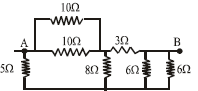Test: Physics - 8 - Question 18

Two charge of magnitude q1 = 2x10-7 C and  q2 = 3x10-7 C are placed 10 cm apart. The electric field produced by  qon  q1 is

Test: Physics - 8 - Question 19

Six resistors each of value 10 Ω are connected to a 5 volt battery as shown in the figure. The reading of ammeter ‘A’ is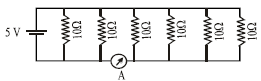Test: Physics - 8 - Question 20

The potential difference between points ‘A’ and‘B  (VB - VA)in the figure if R = 0.7 Ω is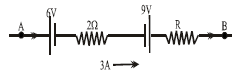Test: Physics - 8 - Question 21
If 2% of the main current is to be passed through the galvanometer of resistance G. the resistance of shunt required is
Test: Physics - 8 - Question 22

If a wire of resistivity ρ  is streched to double its length, then its new resitivity will

Test: Physics - 8 - Question 23

The internal resistance of a primary cell is 4Ω It generates a current of 0.2 A in an external resistance of 21 ΩThe rate at which chemical energy is consumed in providing the current is

Test: Physics - 8 - Question 24

The relation between Faraday constant F, electron charge e, and Avogadro Number N is

Test: Physics - 8 - Question 25

In the circuit, as shown in Fig. The heat produced by 6 Ω resistance due to current flowing in it is 60 calories per second. The heat generated across 3-ohm resistance per second will be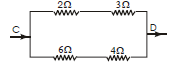Test: Physics - 8 - Question 26

A 100W bulb B1 and two 60W bulb B2 and B3 are connected to a 250 V source as shown in the Fig. Now W1, W2 and W3 are the output powers of the bulbs B1 , B2 and B3 respectively ,Then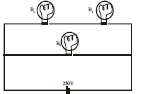Test: Physics - 8 - Question 27

A solenoid 30 cm long is made by winding 2000 loops of wire on an iron rod whose cross section is 1.5 cm2 If the relative permeability of the iron is 600, what is the self inductance of the solenoid

Test: Physics - 8 - Question 28
A thin bar magnet of length 2l and breadth 2b, pole strength p and magnetic moment M is divided into four equal parts with length and breadth of each part being half of the original magnet. Then the pole strength of each part is
Test: Physics - 8 - Question 29
Susceptibility is positive and small for a ........substance:
Test: Physics - 8 - Question 30

A magnetic needle lying parallel to a magnetic field requires W units of work to turn it through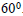The torque needed to maintain the needle in this position
will be

Test: Physics - 8 - Question 31

A charged particle of mass 10-3 kg and charge + 10-5coulomb enters a magnetic field of induction one tesla. If g = 10m/s2for what value of velocity it will pass straight through it without deflection?

Test: Physics - 8 - Question 32
Which one of the following graphs represents correctly the variation of the magnetic field (B) at an axial point,for a circular coil with the distance (x) of the point from the centre of the circular coil?
Test: Physics - 8 - Question 33

A bar magnet of magnetic moment μ is bent to form a semi-circle. Its magnetic moment will become

Test: Physics - 8 - Question 34

At a given place on the earth’s surface the horizontal component of magnetic field is  3.2 x 10-5 tesla and the resultant magnetic field is  6.4 x 10-5 tesla. The angle of dip at that place is:

Test: Physics - 8 - Question 35
The weight of a horizontal current carrying wire can be supported by a magnetic field which is
Test: Physics - 8 - Question 36

A short magnetic needle is pivoted in a uniform magnetic field of strength 1T. When another magnetic field of strength √3 T is applied to the needle in a perpendicular direction, the needle deflects through an angle θ where  θ  is

Test: Physics - 8 - Question 37
The mutual inductance of a pair of coils depends on
Test: Physics - 8 - Question 38
If two bulbs A(25W-220V) & B(100W-220V) are connected in series with the supply of 330 volt, which bulb will fuse?
Test: Physics - 8 - Question 39

An electron moving in a circular orbit of radius R with a period T is equivalent to a magnetic dipole of dipole moment

Test: Physics - 8 - Question 40

Current is flowing in a one turn circular coil. If the coil is bent into a two turn coil of smaller radius, then the magnetic field at the centre of the coil will become

Test: Physics - 8 - Question 41
A rectangular coil of wire having 10 turns with dimensions of 20cmx30cm rotates at a constant speed of 600 rpm in a magnetic field 0.10T. The axis of rotation is perpendicular to the field. The maximum emf produced is (approximately)
Test: Physics - 8 - Question 42

A copper rod moves parallel to the horizontal direction.The induced emf developed across its ends due to earths magnetic field will be maximum at the

Test: Physics - 8 - Question 43

The figure below shows a resistor and a capacitor connected in series with a 25 V rms alternation supply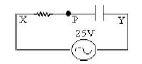What is the r.m.s. potential difference between P and Y given that the r.m.s. potential difference between X and
P is 15 V?

Test: Physics - 8 - Question 44

The equation of an alternating voltage is E = 220 sin  (ωt   a π/6 )nd the equation of the current in the circuit is I = 10 sin (ωt  -  π/6)What is the impedance of the circuit?

Test: Physics - 8 - Question 45

In the circuit shown in Fig. the r.m.s currents I1, I2 and I3, are altered by a varying the frequency ƒ of the oscillator. The output voltage of the oscillator remains sinusoidal and has fixed amplitude.Which curves in Fig.
indicate correctly the variations with frequency of the current I1, I2 and I3?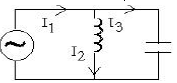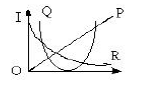## NEET Mock Test Series

1 videos|25 docs|144 tests
 Use Code STAYHOME200 and get INR 200 additional OFF Use Coupon Code
Information about Test: Physics - 8 Page
In this test you can find the Exam questions for Test: Physics - 8 solved & explained in the simplest way possible. Besides giving Questions and answers for Test: Physics - 8, EduRev gives you an ample number of Online tests for practice

## NEET Mock Test Series

1 videos|25 docs|144 tests

### How to Prepare for NEET

Read our guide to prepare for NEET which is created by Toppers & the best Teachers